# Multiplication

Multiplication is the binary mathematical operation of scaling one number or quantity by another (multiplying). It is one of the four basic operations in elementary arithmetic (with addition, subtraction and division). The result of this operation is called product and the multiplied numbers are called factors, or the multiplier and the multiplicand. The product of a and b may be written as a × b or a.b or simply denoted by juxtaposition ab when a and b are symbols or formulae rather than numbers.

The product m × n of a natural number (that is, positive whole number) m times another quantity n agrees with the result of successively adding n to itself a total of m times. For example, 2 multiplied by 3 (often said as "3 times 2") gives the same result as 2 taken 3 times, i.e., of adding 3 copies of 2 : 2 × 3 = 2 + 2 + 2. Similarly, π multiplied by 2 is the same value obtained by adding the number pi to itself.

Multiplication can be visualised as counting objects arranged in a rectangle (for natural numbers) or as finding the area of a rectangle whose sides have given lengths (for positive real numbers generally).

However, multiplication does not give the result of a repeated addition when the multiplier is not a natural number. We can calculate the product$(-\sqrt{2}) \times \sqrt{2} = -2$

using the rules for manipulating radicals and products, but we cannot view this computation as repeatedly adding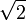$\sqrt{2}$. Instead of being viewed as a special type of repeated addition, multiplication should be viewed as a basic numerical operation, separate from and as important as addition.

The inverse of multiplication is division: as 2 times 3 equals to 6, so 6 divided by 3 equals to 2.

## Properties

Commutativity

Multiplication of numbers is commutative, meaning a × b = b × a.

Associativity

Multiplication is associative, meaning a × (b × c) = (a × b) × c.

Distributivity

Multiplication is distributive over addition, meaning a × (x + y) = a × x + a × y.

## Products of sequences

### Capital pi notation

The product of a sequence can be written using capital Greek letter Π (Pi). Unicode position U+220F (∏) contains a symbol for the product of a sequence, distinct from U+03A0 (Π), the letter. The meaning of this notation is given by: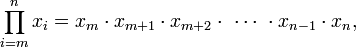$\prod_{i=m}^{n} x_{i} = x_{m} \cdot x_{m+1} \cdot x_{m+2} \cdot \,\,\cdots\,\, \cdot x_{n-1} \cdot x_{n},$

where i is an index of multiplication, m is its lower bound and n is its upper bound. Example: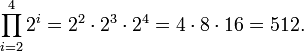$\prod_{i=2}^{4} 2^i = 2^2 \cdot 2^3 \cdot 2^4 = 4 \cdot 8 \cdot 16 = 512.$

If m = n, the value of the product just equals to xm. If m > n, the product is the empty product, with the value 1.

## More general concepts of multiplication

Multiplication can be defined outside of the context of real numbers. There are natural multiplication operations on the complex numbers, matrices, tensors, sequences, and functions.

The properties described need not always hold in these more general settings. In particular, multiplication is not always commutative in other contexts. For example, matrix multiplication is in general not a commutative operation: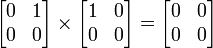$\begin{bmatrix} 0 & 1\\ 0 & 0\\ \end{bmatrix} \times \begin{bmatrix} 1 & 0\\ 0 & 0\\ \end{bmatrix} = \begin{bmatrix} 0 & 0\\ 0 & 0\\ \end{bmatrix}$

but reversing the order of multiplication, we have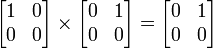$\begin{bmatrix} 1 & 0 \\ 0 & 0 \end{bmatrix} \times \begin{bmatrix} 0 & 1\\ 0 & 0 \end{bmatrix} = \begin{bmatrix} 0 & 1\\ 0 & 0 \end{bmatrix}$

a different result.

A common thread in these examples of operations called "multiplication" is the existence of an additional "addition" operation and a collection of common axioms satisfied by the addition and multiplication operations in each context. This general context in which a multiplication operation exists, encompassing all of the above examples, is that of the abstract ring encountered in abstract algebra.

Multiplication may also be used to denote more general binary operations in groups and semigroups.Some content on this page may previously have appeared on Citizendium.